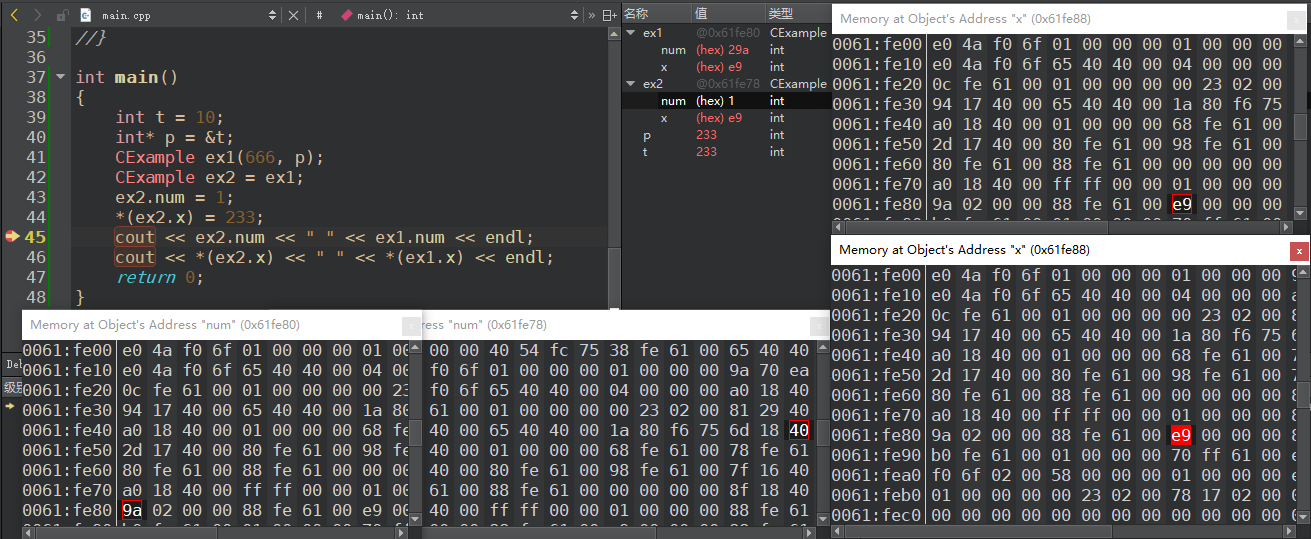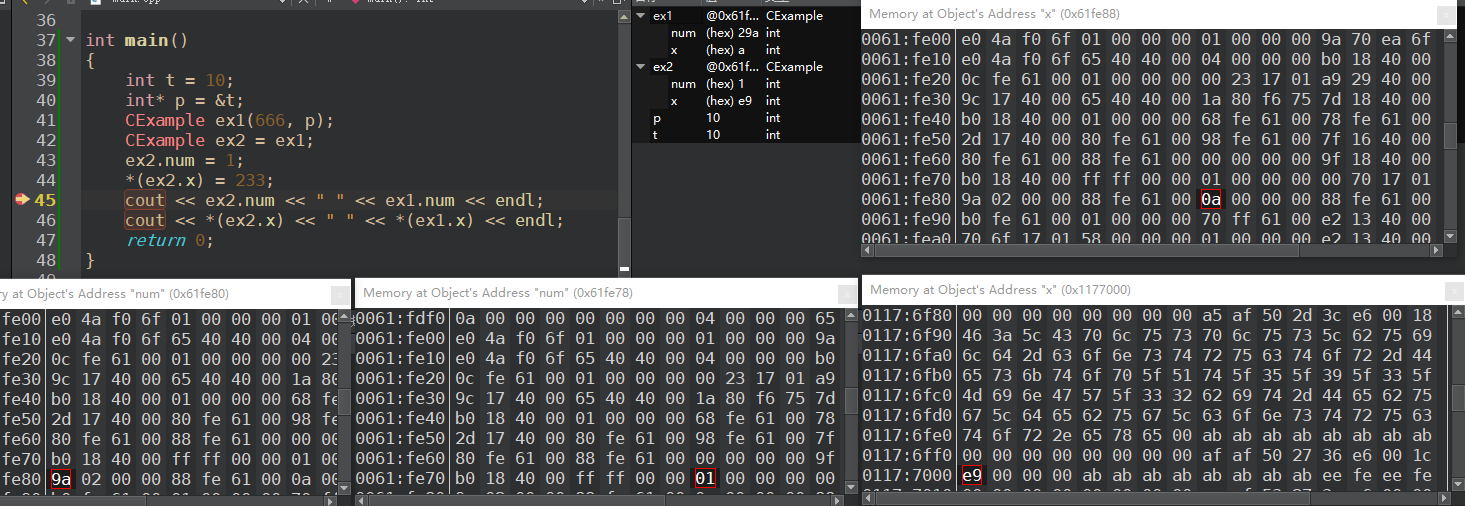# C++ 拷贝构造函数

Posted by kevin on October 13, 2019

## 回顾构造函数

class A{
public:
A(){}	// 默认构造函数
A(int a = 0, int b = 0){}	// 带默认参数的构造函数
A(int a, int b, int c){}	// 带参数构造函数
};


## 拷贝构造函数

int a = 0;
int b = a;


class CExample {
public:
CExample(int b)
{
a = b;
}

void Show ()
{
cout<<a<<endl;
}
private:
int a;
};

int main()
{
CExample A(10);
CExample B = A; // 对象初始化要调用拷贝构造函数
B.Show ();
return 0;
}


CExample(const CExample & B){
a = B.a;
}


## 调用拷贝构造函数的时机

### 0x01 对象以值传递的方式传入函数参数

class CExample {
public:
//构造函数
CExample(int b)
{
a = b;
cout << "creat:" << a << endl;
}

//拷贝构造
CExample(const CExample& C)
{
a = C.a;
cout << "copy" << endl;
}

//析构函数
~CExample()
{
cout << "delete:" << a << endl;
}

private:
int a;
};

void g_Fun(CExample C)
{
cout<<"test"<<endl;
}

int main()
{
CExample test(1);
g_Fun(test);
return 0;
}


main 函数调用 g_Fun() 函数时的步骤如下：

1. test 对象传入形参，会产生一个临时变量 C
2. 调用拷贝构造函数把 test 的值给 C，前面两步有点像 CExample C(test)
3. 执行完 g_Fun() ，调用析构函数析构 C 对象

creat: 1
copy
test
delete: 1
delete: 1


### 0x02 对象以值传递的方式从函数返回

CExample g_Fun()
{
CExample temp(0);
return temp;
}

int main()
{
g_Fun();
return 0;
}


1. 产生一个临时变量 C
2. 调用拷贝构造函数将 temp 的值复制给 C ，前面两步像 CExample C(temp)
3. 在函数执行到最后先调用析构函数析构 temp 局部变量
4. g_Fun() 执行完后再析构掉 C 对象

creat: 0
delete: 0


### 0x03 对象需要通过另外一个对象进行初始化

CExample A(10);
CExample B = A;
// CExample B(A);


creat: 10
copy
delete: 10
delete: 10



## 深拷贝和浅拷贝

### 浅拷贝

Rect::Rect(const Rect& r)
{
width = r.width;
height = r.height;
}


class Rect
{
public:
Rect()      // p指向 堆 中分配的空间
{
p = new int(100);
}
~Rect()     // 释放动态分配的空间
{
if(p != NULL)
{
delete p;
}
}
private:
int width;
int height;
int *p;
};

int main()
{
Rect rect1;
Rect rect2(rect1);
return 0;
}


### 深拷贝

class Rect
{
public:
Rect()
{
p = new int(100);
}
Rect(const Rect& r)
{
width = r.width;
height = r.height;
p = new int;    // 为新对象重新动态分配空间
*p = *(r.p);
}
~Rect()
{
if(p != NULL)
{
delete p;
}
}
private:
int width;
int height;
int *p;
};


## FAQ

### 0x01 以下函数哪个是拷贝构造函数

X::X(const X&);
X::X(X);
X::X(X&, int a=1);
X::X(X&, int a=1, int b=2);


• X &
• const X &
• volatile X &
• const volatile X &

### 0x02 一个类中可以存在多于一个的拷贝构造函数吗

class X {
public:
X();
X(X&);
};

const X cx;
X x = cx;


## 实验时间

class CExample
{
public:
int num;
int *x;

public:
//构造函数
CExample(int b, int * ss)
{
num = b;
x = ss;
}

//拷贝构造
CExample(const CExample& C)
{
num = C.num;
x = C.x;
cout<<"copy"<<endl;
}
};

int main()
{
int t = 10;
int* p = &t;
CExample ex1(666, p);
CExample ex2 = ex1;
ex2.num = 1;
*(ex2.x) = 233;
cout << ex2.num << " " << ex1.num << endl;
cout << *(ex2.x) << " " << *(ex1.x) << endl;
return 0;
}CExample(const CExample& C)
{
num = C.num;
x = new int;
*x = *(C.x);
cout<<"copy"<<endl;
}## reference

https://blog.csdn.net/sxhelijian/article/details/50977946